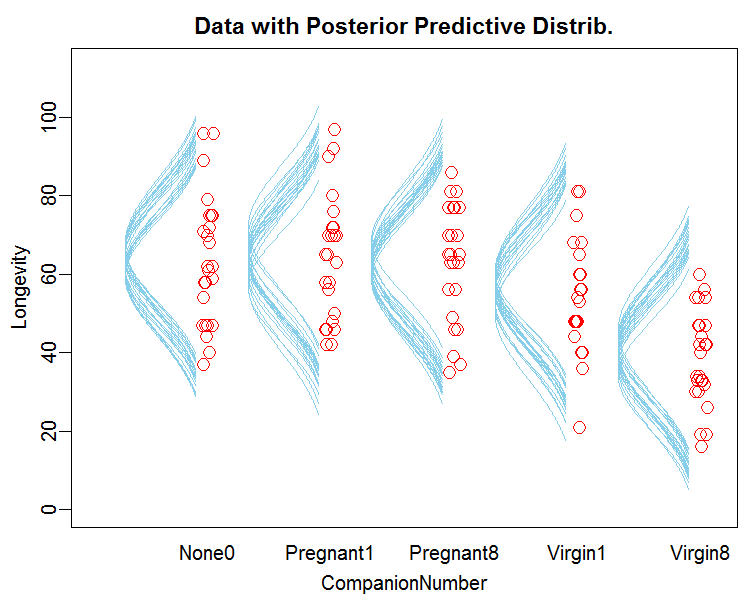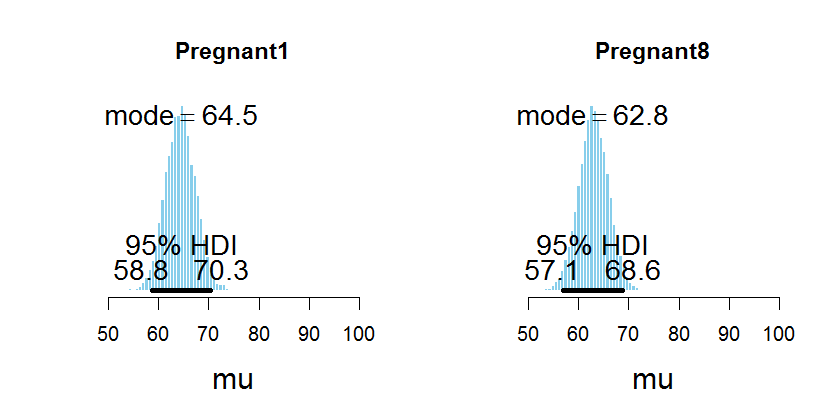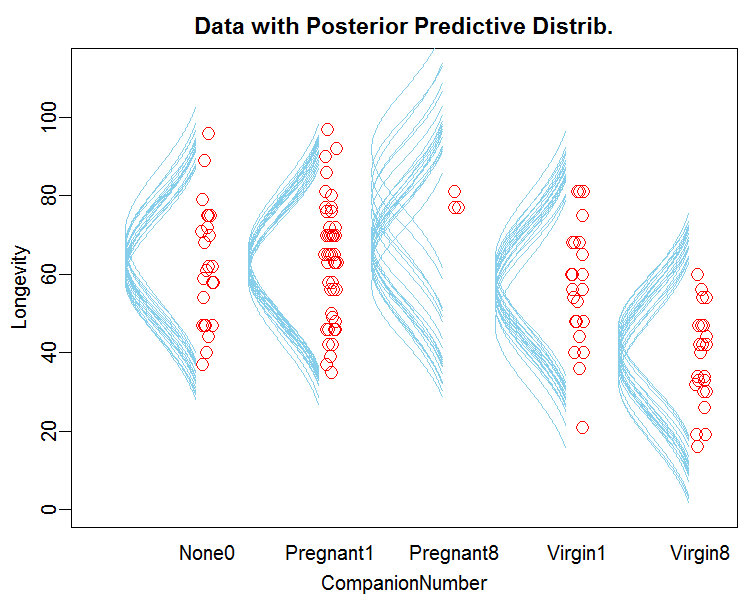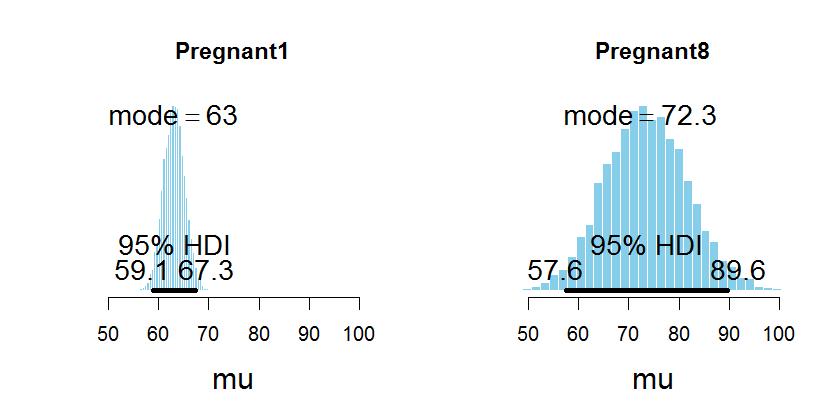## Tuesday, April 7, 2015

### Sex, death, and unbalanced designs (done Bayesian)

In classical analysis of variance (ANOVA), data with unequal samples sizes in the different cells can be a headache. These are often called "unbalanced" designs. But Bayesian analysis works identically for unbalanced designs as for balanced designs. This post shows an example. We'll see that the small-N cells naturally have less precise estimates and more shrinkage than the large-N cells.

Consider a study of the life span (i.e., longevity measured in days) of male subjects as a function of how much sexual activity they endured. The males were put into five different treatment groups, each group allowed different levels of sexual activity. You can look in Section 19.3.2 of DBDA2E, p. 561, for more details, but one more detail is that the species being studied was the fruit fly.

The analysis of the original balanced design yields this result (as in Figure 19.3, p. 563):In particular, here are the estimates of the means for Pregnant1 and Pregnant8:Notice above that the widths of the HDIs are about the same (i.e., about 11 days). The widths are about the same because (well, at least in part because) the two groups have the same amount of data (N=25).

Now we will unbalance the design by arbitrarily shifting most of the Pregnant8 data into the Pregnant1 group. Using the identical analysis program, with no changes except to the data, here is the result:Notice above that the HDI for mu of Pregnant1 is narrower than before, because the sample size for that cell is now larger, while the HDI for mu of Pregnant8 is wider than before, because the sample size for that cell is now smaller.

Notice also the shrinkage on mu for Pregnant8: Although the data (see the figure) have a sample mean near 80, the estimated mu has a mode near 72. The shrinkage is caused by the hierarchical structure of the model.

In summary, it's neat that Bayesian models don't care about whether there are equal sample sizes in the cells. The model works the same way regardless, and the posterior distribution makes sense: The cell with small sample size has greater uncertainty and more shrinkage.

Appendix: Code for generating the results above.

The basic analysis is from the script Jags-Ymet-Xnom1grp-Mnormal-Example.R. To generate the graphs of mu, I added the following to the very end of the script:

mcmcMat = as.matrix( mcmcCoda )
p1idx = which(levels(myDataFrame[,xName])=="Pregnant1")
p8idx = which(levels(myDataFrame[,xName])=="Pregnant8")
openGraph(width=7,height=3.5)
layout(matrix(1:2, nrow=1))
plotPost( mcmcMat[,paste0("m[",p1idx,"]")] , main="Pregnant1" , xlab="mu" , xlim=c(50,100) )
plotPost( mcmcMat[,paste0("m[",p8idx,"]")] , main="Pregnant8" , xlab="mu" , xlim=c(50,100) )

To recode the group labels of the data, I used the following extra lines immediately after the data file is read in near the top of the script:

# Take lots of data out of Pregnant8 and recode it as Pregnant1:
p1 = myDataFrame[,"CompanionNumber"]=="Pregnant1"
p8 = myDataFrame[,"CompanionNumber"]=="Pregnant8"
myDataFrame[p8,"CompanionNumber"][1:22] = "Pregnant1"

1.Dear Prof,

what about if my data are unbalanced by choice and one of the levels of a factor is completely missing observations? Should I correct the model structure, for example setting the interaction for that level factor with other factor levels to 0? Should I re-adapt the sum to 0 par too?

Best,
Matteo

2.Well, if you have a very small design with empty cells, it would probably be better to reconceptualize the factors; e.g., a 2x2 with one empty cell would be better to treat as a 3-group design because you can't really estimate the main effects and interaction.

But a larger design with missing cells is no problem. Chapter 20 of DBDA2E has an extended example; see Figure 20.3

3.Can this be implemented in a glm setting? I would like to run an analysis were some predictors (lets say x1 and x2) have the same replication as the response (y) but other predictors (x3) has a different level of replication.

Thanks!

1.DBDA2E has an example of a split-plot design (1 between-subject factor, 1 within-subject factor). For complex designs, try the brms package for Stan.

2.Thanks. Perhaps I am showing my naiveté, but I read that section and I think I see what it its getting at, but in my case all the data (x1, x2, x3, y) can be attributed to the same "site". Its just that y, x1, and x2 are data that were collected with 8 replicates per site and x3 was collected with 3. Can the a split plot approach still help me? I should also note that there are multiple sites that I am already treating "site" as a random effect in the glm.

3.I think you may need to consult someone in person to understand the design of your data. From your brief description, it seems you may have enough data at each site that you could model each site with its own parameters, and then include hierarchical structure to estimate what's typical across sites.

4.Dear Prof. Kruschke

I am having similar problem of a missing level in one of the factors. My design is 2 factors (1st 2 levels and 2nd with 3 levels) tested across 3 diff populations. Can I convert the 2*3 design to a 5 group design and run it as repeated measures ANOVA?

Thank you

Alekhya

1.Sure, you can re-conceptualize the factor structure of the groups. (You can do that in traditional frequentist analysis too, of course.)

As an aside, in Section 20.2.2 of DBDA2E there's an example of a 2-factor structure with lots of missing cells.

2.Dear Prof. Kruschke